## How to Calculate and Solve for Total Compressibility of a Reservoir Fluid Flow | The Calculator Encyclopedia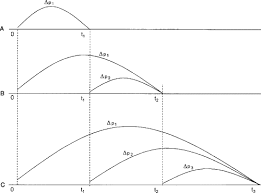The image above represents the total compressibility.

To compute for the total compressibility, seven essential parameters are needed and these parameters are Water compressibility (Cw), Oil compressibility (Co), Gas compressibility (Cg), Rock compressibility (Cr), water saturation (Sw), Oil saturation (So) and Gas saturation (Sg).

The formula for calculating the total compressibility:

CT = CoSo + CgSg + CwSw + Cr

Where;

CT = Total Compressibility
Cw = Water Compressibility
Co = Oil Compressibility
Cg = Gas Compressibility
Cr = Rock Compressibility
Sw = Water Saturation
So = Oil Saturation
Sg = Gas Saturation

Let’s solve an example;
With a given value of 13 as water compressibility, 17 as oil compressibility, 19 as gas compressibility, 25 as rock compressibility, 24 as water saturation, 29 as oil saturation and 21 as gas saturation.
Find the total compressibility?

This implies that;

Cw = Water Compressibility = 13
Co = Oil Compressibility = 17
Cg = Gas Compressibility = 19
Cr = Rock Compressibility = 25
Sw = Water Saturation = 24
So = Oil Saturation = 29
Sg = Gas Saturation = 21

CT = CoSo + CgSg + CwSw + Cr
CT = (17 x 29) + (19 x 21) + (13 x 24) + 25
CT = 493 + 399 + 312 + 25
CT = 1229

Therefore, the total compressibility is 1229 psi.

## How to Calculate and Solve for Reservoir Radius and Well Spacing | The Calculator Encyclopedia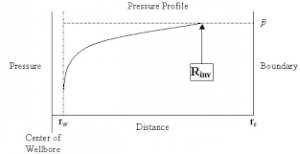The image above represents the reservoir radius.

To compute for the reservoir radius, one essential parameter is needed and this parameter is Well Spacing (A).

The formula for calculating the reservoir radius:

re = √[43560A  / π]

Where;

A = Well Spacing

Let’s solve an example;
Given that the well spacing of an reservoir radius is 42. Find the reservoir radius?

This implies that;

A = Well Spacing = 42

re = √[43560A  / π]
re = √[43560 x 42 / π]
re = √[1829520 / π]
re = √582354.3
re = 763.12

Therefore, the reservoir radius is 763.12 ft.

## How to Calculate and Solve for Linear Flow Rate in Reservoir Fluid Flow | The Calculator Encyclopedia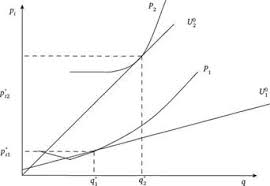The image above represents the linear flow rate.

To compute for the linear flow rate, four parameters are needed and these parameters are Initial pressure (P1), Final Pressure (P2), Thickness (h) and Permeability (k).

The formula for calculating linear flow rate:

q = 0.001127kh[P1 – P2]

Where:
q = Linear Flow Rate
P1 = Initial Pressure
P2 = Final Pressure
h = Thickness
k = Permeability

Let’s solve an example;
Find the linear flow rate when the initial pressure is 12, final pressure is 22, thickness is 18 and permeability is 44.

This implies that;
P1 = Initial Pressure = 12
P2 = Final Pressure = 22
h = Thickness = 18
k = Permeability = 44

q = 0.001127kh [P1 – P2]
q = 0.001127 x 44 x 18 [12 – 22]
q = 0.001127 x 44 x 18 [-10]
q = 0.892584 [-10]
q = -8.92584

Therefore, the linear flow rate is -8.92584 bbl/day.

Calculating Permeability when the linear flow rate, Initial Pressure, Final Pressure and Thickness is Given.

k = q / 0.001127h (p1 – p2)

Where;
k = Permeability
q = Linear Flow Rate
P1 = Initial Pressure
P2 = Final Pressure
h = Thickness

Let’s solve an example;
Find the permeability when the initial pressure is 34, final pressure is 24, thickness is 12 and linear flow rate is 50.

This implies that;
q = Linear Flow Rate = 50
P1 = Initial Pressure = 34
P2 = Final Pressure = 24
h = Thickness = 12

k = q / 0.001127h (p1 – p2)
k = 50 / 0.001127h (34 – 24)
k = 50 / 0.001127h (10)
k = 50 / 0.01127
k = 4436.5

Therefore, the permeability is 4436.5.

## How to Calculate and Solve for Fluid Potential, Pressure, Datum Level and Density | The Calculator Encyclopedia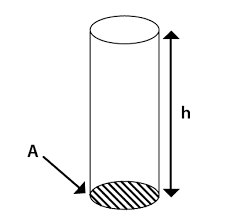The image above represents the fluid potential.

To compute for the fluid potential, three essential parameters are needed and these parameters are pressure (P), Datum Levels (ΔZ) and Density (ρ).

The formula for calculating fluid potential:

φ = P – [ρ / 144]ΔZ

Where;
φ = Fluid Potential
P = Pressure
ΔZ = Datum Levels
ρ = Density

Let’s solve an example;
Find the fluid potential when the pressure is 24 with a datum level of 18 and the density of 30.

This implies that;
P = Pressure = 24
ΔZ = Datum Levels = 18
ρ = Density = 30

φ = P – [ρ / 144]ΔZ
φ = 24 – [30/144] 18
φ = 24 – [0.2083] 18
φ = 24 – 3.75
φ = 20.25

Therefore, the fluid potential is 20.25.

Calculating the Pressure(P) when the fluid potential, Datum levels and Density is Given.

P = φ – [ρ / 144]ΔZ

Where;
P = Pressure
φ = Fluid Potential
ΔZ = Datum Levels
ρ = Density

Lets solve an example;
Find the pressure with a fluid potential of 40 and a datum levels of 18 with density of 24.

This implies that;
φ = Fluid Potential = 40
ΔZ = Datum Levels = 18
ρ = Density = 24

P = φ – [ρ / 144]ΔZ
P = 40 – [24 / 144]18
P = 40 – [0.167]18
P = 40 – 3
P = 37

Therefore, the pressure is 37.

## How to Calculate and Solve for True Stress | The Calculator Encyclopedia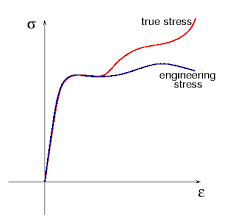The image above represents the true stress.

To compute for the true stress, two essential parameters are needed and these parameters are force (F) and instantaneous area (Ai).

The formula for calculating true stress:

σT = F / Ai

Where;
T = True Stress
F = Force
Ai = Instantaneous Area

Let’s solve an example;
Find the true stress when the instantaneous area is 60 with a force of 25.

This implies that;
F = Force = 25
Ai = Instantaneous Area = 60

σT = F / Ai
σT = 25 / 60
σT = 0.416

Therefore, the true stress is 0.416 Pa.

Calculating the Force when True Stress and Instantaneous Area is Given.

F = Ai x σT

Where;
F = Force
σT = True Stress
Ai = Instantaneous Area

Let’s solve an example;
Find the force when the instantaneous area is 30 with a true stress of 15.

This implies that;
σT = True Stress = 15
Ai = Instantaneous Area = 30

F = Ai x σT
F = 30 x 15
F = 450

Therefore, the force is 450.

## How to Calculate and Solve for Road Bank Angle, Velocity and Radius of a Body in Motion of Circular Path | The Calculator Encyclopedia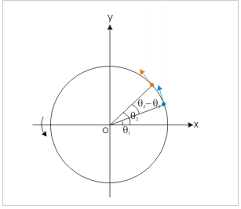The image represents road bank angle in circular motion.

To compute for the road bank angle, three essential parameters are needed and these parameters are velocity (v), acceleration due to gravity (g) and radius (r).

The formula for calculating the road bank angle;

θ = tan-1( / gr)

Where;
v = Velocity
g = Acceleration due to Gravity

Let’s solve an example;
Find the road bank angle where the acceleration due to gravity is 9.8, velocity is 35 and radius is 18.

This implies that;
v = Velocity = 35
g = Acceleration due to Gravity = 9.8

θ = tan-1( / gr)
θ = tan-1(35² / (9.8)(18))
θ = tan-1(1225 / 176.4)
θ = tan-1(6.94)
θ = 81.81°

Therefore, the road bank angle is 81.81°.

Calculating the Velocity when Road Bank Angle, Acceleration due to Gravity and Radius is Given.

v = √gr.tan θ

Where;
v = Velocity
g = Acceleration due to Gravity

Let’s solve an example;
Given that the road bank angle is 50, radius is 15 and acceleration due to gravity is 9.8. Find the velocity?

This implies that;
θ = Road Bank Angle = 50
g = Acceleration due to Gravity = 9.8

v = √gr.tan θ
v = √(9.8 x 15)(tan 50)
v = √(147)(1.1917)
v = √175.1799
v = 13.235

Therefore, the velocity is 13.235.

## How to Calculate and Solve for Centripetal Acceleration, Angular Velocity and Radius of Circular Path of a Body | The Calculator Encyclopedia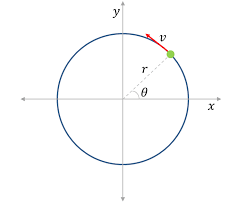The image above represents centripetal acceleration in a motion of circular path.

To compute for the centripetal acceleration, two essential parameters are needed and these parameters are angular velocity (ω) and radius of circular path (r).

The formula for the calculating centripetal acceleration:

a = ω²r

Where:
a = Centripetal Acceleration
ω = Angular Velocity
r = Radius of Circular Path

Let’s solve an example;
Find the centripetal acceleration with an angular velocity of 33 and a radius of 21.

This implies that;
ω = Angular Velocity = 33
r = Radius of Circular Path = 21

a = ω²r
a = 33² x 21
a = 1089 x 21
a = 22869

Therefore, the centripetal acceleration is 22869 m/s².

Calculating the Angular Velocity when Centripetal Acceleration and Radius of Circular path is Given.

ω = √a / r

Where:
ω = Angular Velocity
a = Centripetal Acceleration
r = Radius of Circular Path

Let’s solve an example;
Find the angular velocity with a centripetal acceleration of 320 and a radius of 8.

This implies that;
a = Centripetal Acceleration = 320
r = Radius of Circular Path = 8

ω = √a / r
ω = √320 / 8
ω = √40
ω = 6.32

Therefore, the angular velocity is 6.32.

## How to Calculate and Solve for Maximum Velocity, Coefficient of Friction and Radius of a Body in Motion of Circular Path | Nickzom Calculator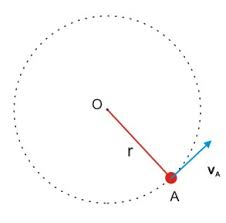The image above represents maximum velocity in circular motion.

To compute for the maximum velocity, three essential parameters are needed and these parameters are coefficient of friction (μ), radius (r) and acceleration due to gravity (g).

The formula for calculating maximum velocity:

Vmax = √(μgr)

Where;
Vmax = maximum velocity
μ = coefficient of friction
g = acceleration due to gravity

Let’s solve an example;
Find the maximum velocity when the coefficient of friction is 14 with a radius of 7 and acceleration due to gravity of 9.8.

This implies that;
μ = coefficient of friction = 14
g = acceleration due to gravity = 9.8

Vmax = √(μgr)
Vmax = √(14 x 7 x 9.8)
Vmax = √(960.40)
Vmax = 30.99

Therefore, the maximum velocity is 30.99 m/s.

Calculating the Coefficient of Friction when the Maximum Velocity, Radius and Acceleration due to Gravity is Given.

μ = Vmax2 / gr

Where;
μ = coefficient of friction
Vmax = maximum velocity
g = acceleration due to gravity

Let’s solve an example;
Find the coefficient of friction with a maximum velocity of 120, radius of 15 and acceleration due to gravity is 9.8?

This implies that;
Vmax = maximum velocity = 120
g = acceleration due to gravity = 9.8

μ = Vmax2 / gr
μ = 1202 / 15 x 9.8
μ = 14400 / 147
μ = 97.96

Therefore, the coefficient of friction is 97.96.

## How to Calculate and Solve for Number of Revolutions per Minute and Angular Velocity of Motion of Circular Path | The Calculator Encyclopedia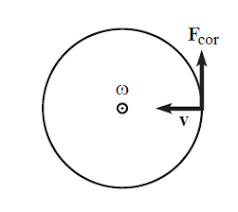The image above represent angular velocity.

To compute the angular velocity, one essential parameter is needed and its parameter is Number of Revolutions per Minute (N).

The formula for calculating angular velocity:

ω = 2πN / 60

Where;
ω = Angular velocity
N = Number of revolutions per minute

Let’s solve an example;
Find the Angular Velocity with a number of revolutions per minute as 60.

This implies that;
N = Number of revolutions per minute = 60

ω = 2πN / 60
ω = 2 x π x 24 / 60
ω = 150.816/ 60
ω = 2.5136

Therefore, the angular velocity is 2.5136 rad/s.

Calculating the Number of Revolutions per Minute when Angular Velocity is Given.

N = ω60 /

Where;
N = Number of revolutions per minute
ω = Angular velocity

Let’s solve an example;
With an angular velocity of 40. Find the number of revolutions per minute?

This implies that;
ω = Angular velocity = 40

N = ω60 /
N = 40 x 60 / 6.284
N = 2400 / 6.284
N = 381.9

Therefore, the number of revolutions per minute is 381.9 min.

## How to Calculate and Solve for Mass, Angular Velocity, Radius and Centrifugal Force of a Body | The Calculator Encyclopedia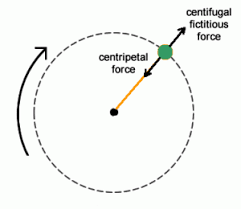The image above represents the centrifugal force.

To compute for the centrifugal force, three essential parameters are needed and these parameters are Mass of the body (m), Angular Velocity of the body (w) and Radius (r).

The formula for calculating the centrifugal force:

F = mω²r

Where:
F = Centrifugal Force
m = mass of the body
ω = angular velocity

Let’s solve an example;
Find the centrifugal force with mass of the body as 12, angular velocity as 32 and a radius of 8.

This implies that;
m = mass of the body = 12
ω = angular velocity = 32

F = mω²r
F = 12 x 32² x 8
F = 12 x 1024 x 8
F = 98304

Therefore, the centrifugal force is 98304 N.

Calculating the Mass of the body (m) when the Centrifugal Force, Angular Velocity and Radius is Given.

m = F / w2r

Where;
m = mass of the body
F = Centrifugal Force
ω = angular velocity

Let’s solve an example;
Find the mass of a body when centrifugal force is 140 with an angular velocity of 24 and a radius of 10.

This implies that;
F = Centrifugal Force = 140
ω = angular velocity = 24